• C++ 中数组很坑，有没有类似 Python 中 list 的数据类型呢？类似的就是 vectorvector 是同一种类型的对象的集合，每个对象都有一个对应的整数索引值。和 string 对象一样，标准库将负责管理与存储元...


在 c++ 中，vector 是一个十分有用的容器。它能够像容器一样存放各种类型的对象，简单地说，vector是一个能够存放任意类型的动态数组，能够增加和压缩数据。C++ 中数组很坑，有没有类似 Python 中 list 的数据类型呢？类似的就是 vector！vector 是同一种类型的对象的集合，每个对象都有一个对应的整数索引值。和 string 对象一样，标准库将负责管理与存储元素相关的内存。我们把 vector 称为容器，是因为它可以包含其他对象。一个容器中的所有对象都必须是同一种类型的。使用vector注意事项：1、如果你要表示的向量长度较长(需要为向量内部保存很多数)，容易导致内存泄漏，而且效率会很低；2、Vector 作为函数的参数或者返回值时，需要注意它的写法：double Distance(vector<int>&a, vector<int>&b) 其中的“&”绝对不能少！！！vector对象的定义和初始化同样的，使用前，导入头文件 #include 可以使用using声明：using std::vector;vector 是一个类模板(class template)。使用模板可以编写一个类定义或函数定义，而用于多个不同的数据类型。因此，我们可以定义保存 string 对象的 vector，或保存 int 值的 vector，又或是保存自定义的类类型对象(如 Sales_items 对象)的 vector。声明从类模板产生的某种类型的对象，需要提供附加信息，信息的种类取决于模板。以 vector 为例，必须说明 vector 保存何种对象的类型，通过将类型放在类型放在类模板名称后面的尖括号中来指定类型：vector v1;保存类型为 T 对象。默认构造函数 v1 为空。vector v2(v1); v2 是 v1 的一个副本。vector v3(n, i);v3 包含 n 个值为 i 的元素。vector v4(n); v4 含有值初始化的元素的 n 个副本。【注意：1、若要创建非空的 vector 对象，必须给出初始化元素的值；2、当把一个 vector 对象复制到另一个 vector 对象时，新复制的 vector 中每一个元素都初始化为原 vectors 中相应元素的副本。但这两个 vector 对象必须保存同一种元素类型；3、可以用元素个数和元素值对 vector 对象进行初始化。构造函数用元素个数来决定 vector 对象保存元素的个数，元素值指定每个元素的初始值】vector对象动态增长：vector 对象(以及其他标准库容器对象)的重要属性就在于可以在运行时高效地添加元素。注意：因为 vector 增长的效率高，在元素值已知的情况下，最好是动态地添加元素。实例：vectortest;//建立一个vector，int为数组元素的数据类型，test为动态数组名简单的使用方法如下：vectortest;//建立一个vectortest.push_back(1);test.push_back(2);//把1和2压入vector，这样test就是1,test就是2实例：vector > points; //定义一个二维数组points.size();  //指第一行的列数1 、基本操作(1)头文件#include.(2)创建vector对象，vector vec;(3)尾部插入数字：vec.push_back(a);(4)使用下标访问元素，cout<(5)使用迭代器访问元素.vector::iterator it;for(it=vec.begin();it!=vec.end();it++)    cout<(6)插入元素：vec.insert(vec.begin()+i,a); 在第i+1个元素前面插入a;(7)删除元素：vec.erase(vec.begin()+2); 删除第3个元素vec.erase(vec.begin()+i,vec.end()+j); 删除区间[ i,j-1] 区间从0开始(8)向量大小: vec.size();(9)清空: vec.clear();特别提示：这里有 begin() 与 end() 函数、front() 与 back() 的差别2、重要说明vector 的元素不仅仅可以是 int,double,string 还可以是结构体，但是要注意：结构体要定义为全局的，否则会出错。#include#include#include#includeusing namespace std;typedef struct rect{    int id;    int length;    int width;　　//对于向量元素是结构体的，可在结构体内部定义比较函数，下面按照id,length,width升序排序。　　bool operator< (const rect &a)  const      {        if(id!=a.id)            return id        else          {            if(length!=a.length)                return length            else                  return width        }    }}Rect;int main(){    vector vec;    Rect rect;    rect.id=1;    rect.length=2;    rect.width=3;    vec.push_back(rect);    vector::iterator it=vec.begin();    cout<' '<' '<endl;  return 0;}3、算法(1) 使用reverse将元素翻转：需要头文件 #includereverse(vec.begin(),vec.end());将元素翻转，即逆序排列！(在vecto r中，如果一个函数中需要两个迭代器，一般后一个都不包含)(2)使用 sort 排序：需要头文件 #include，sort(vec.begin(),vec.end());(默认是按升序排列,即从小到大).可以通过重写排序比较函数按照降序比较，如下：定义排序比较函数：bool Comp(const int &a,const int &b){return a>b;}调用时: sort(vec.begin(),vec.end(),Comp)，这样就降序排序。 输出Vector的中的元素   vector vecClass; int nSize = vecClass.size();    //打印 vecClass,方法一： for(int i=0;i{   cout<"     ";}   cout<需要注意的是：以方法一进行输出时，数组的下表必须保证是整数。 //打印 vecClass,方法二：    for(int i=0;i{   cout<"     ";}   cout<//打印 vecClass,方法三：输出某一指定的数值时不方便for(vector::iterator it = vecClass.begin();it!=vecClass.end();it++){    cout<"   ";}    cout<二维数组的使用：#include "stdafx.h"  #include #include #include using namespace std;int main(){    using namespace std;    int out = { 1, 2,             3, 4,            5, 6 };    vector <int*> v1;    v1.push_back(out);    v1.push_back(out);    v1.push_back(out);    cout << v1 << endl;//1    cout << v1 << endl;//2    cout << v1 << endl;//3    cout << v1 << endl;//4    cout << v1 << endl;//5    cout << v1 << endl;//6    return 0;}展开全文• 因子分析(factor analysis)因子分析的一般步骤factor_analyzer模块进行因子分析使用Python实现因子分析初始化构建数据将原始数据标准化处理 X计算相关矩阵C计算相关矩阵C的特征值和特征向量确定公共因子个数k构造...


因子分析(factor analysis)因子分析的一般步骤factor_analyzer模块进行因子分析使用Python实现因子分析初始化构建数据将原始数据标准化处理 X计算相关矩阵C计算相关矩阵C的特征值  和特征向量   确定公共因子个数k构造初始因子载荷矩阵A建立因子模型将因子表示成变量的线性组合.计算因子得分.因子分析(factor analysis)是指研究从变量群中提取共性因子的统计技术。因子分析是简化、分析高维数据的一种统计方法。因子分析又存在两个方向，一个是探索性因子分析(exploratory factor analysis)。另一个是验证性因子分析(confirmatory factor analysis)。探索性因子分析是先不假定一堆自变量背后到底有几个因子以及关系，而是我们通过这个方法去寻找因子及关系。验证性因子分析是假设一堆自变量背后有几个因子，试图验证这种假设是否正确。因子分析有两个核心问题，一是如何构造因子变量，二是如何对因子变量进行命名解释。因子分析的一般步骤将原始数据标准化处理 X计算相关矩阵C计算相关矩阵C的特征值 r 和特征向量 U确定公共因子个数k构造初始因子载荷矩阵,其中U为r的特征向量建立因子模型对初始因子载荷矩阵A进行旋转变换，旋转变换是使初始因子载荷矩阵结构简化，关系明确，使得因子变量更具有可解释性，如果初始因子不相关，可以用方差极大正交旋转，如果初始因子间相关，可以用斜交旋转，经过旋转后得到比较理想的新的因子载荷矩阵A'.将因子表示成变量的线性组合，其中的系数可以通过最小二乘法得到.计算因子得分.factor_analyzer模块进行因子分析算法核心：对若干综合指标进行因子分析并提取公共因子,再以每个因子的方差贡献率作为权数与该因子的得分乘数之和构造得分函数。import pandas as pdimport numpy as npfrom pandas import DataFrame,Seriesfrom factor_analyzer import FactorAnalyzerimport warningswarnings.filterwarnings("ignore")使用Python实现因子分析初始化构建数据 #构建数据集data=pd.DataFrame(np.random.randint(50,100,size=(5, 10)))data.columns=["特征1","特征2","特征3","特征4","特征5","特征6","特征7","特征8","特征9","特征10"]data.index=['对象1','对象2','对象3','对象4','对象5']#查看数据data.head(3)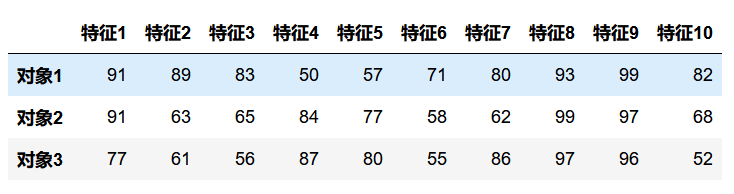将原始数据标准化处理 X data=(data-data.mean())/data.std() # 0均值规范化data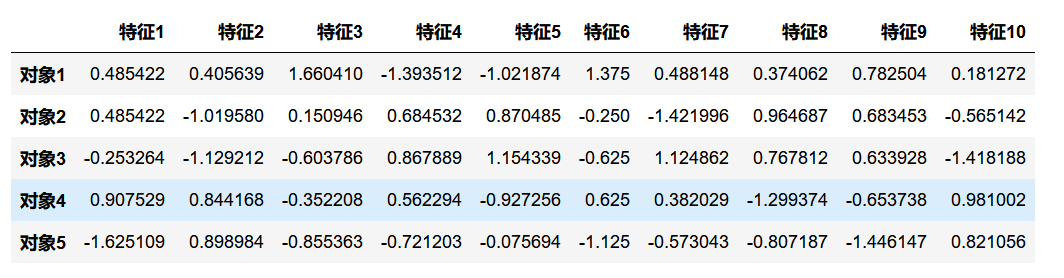计算相关矩阵C C=data.corr() #相关系数矩阵C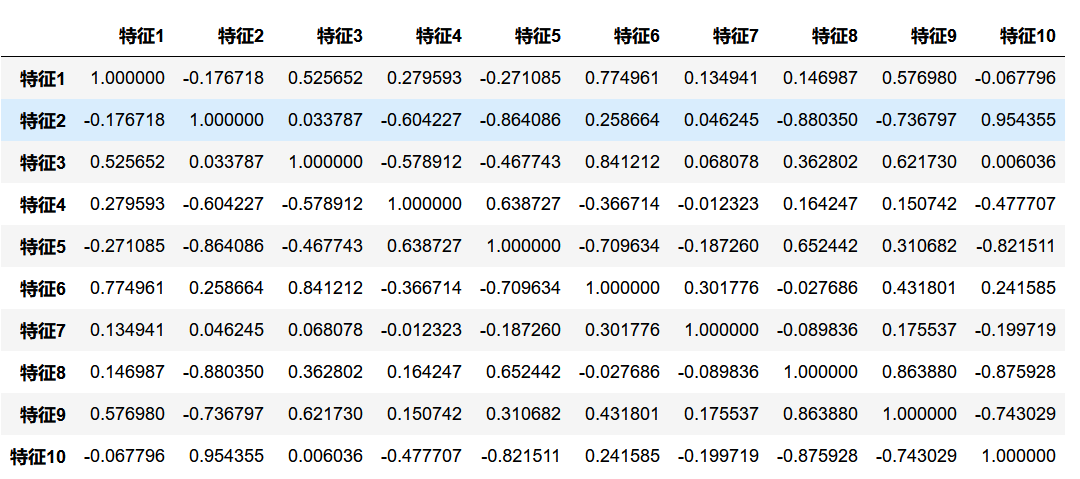计算相关矩阵C的特征值    和特征向量    import numpy.linalg as nlg #导入nlg函数，linalg=linear+algebraeig_value,eig_vector=nlg.eig(C) #计算特征值和特征向量eig=pd.DataFrame() #利用变量名和特征值建立一个数据框eig['names']=data.columns#列名eig['eig_value']=eig_value#特征值eig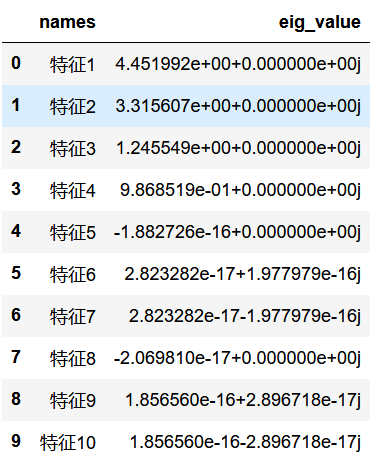确定公共因子个数k from math import sqrtfor k in range(1,11): #确定公共因子个数    if eig['eig_value'][:k].sum()/eig['eig_value'].sum()>=0.8: #如果解释度达到80%, 结束循环        print(k)        break3eig['eig_value'][:3].sum()/eig['eig_value'].sum()(0.9013148087274826+0j)构造初始因子载荷矩阵A col0=list(sqrt(eig_value)*eig_vector[:,0]) #因子载荷矩阵第1列col1=list(sqrt(eig_value)*eig_vector[:,1]) #因子载荷矩阵第2列col2=list(sqrt(eig_value)*eig_vector[:,2]) #因子载荷矩阵第3列A=pd.DataFrame([col0,col1,col2]).T #构造因子载荷矩阵AA.columns=['factor1','factor2','factor3'] #因子载荷矩阵A的公共因子A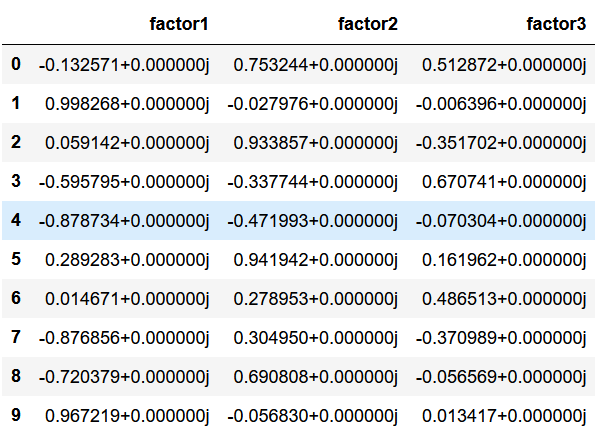建立因子模型 h=np.zeros(10) #变量共同度，反映变量对共同因子的依赖程度，越接近1，说明公共因子解释程度越高，因子分析效果越好D=np.mat(np.eye(10))#特殊因子方差，因子的方差贡献度 ，反映公共因子对变量的贡献，衡量公共因子的相对重要性A=np.mat(A) #将因子载荷阵A矩阵化for i in range(10):    a=A[i,:]*A[i,:].T #行平方和    h[i]=a[0,0]  #计算变量X共同度,描述全部公共因子F对变量X_i的总方差所做的贡献，及变量X_i方差中能够被全体因子解释的部分    D[i,i]=1-a[0,0] #因为自变量矩阵已经标准化后的方差为1，即Var(X_i)=第i个共同度h_i + 第i个特殊因子方差将因子表示成变量的线性组合. from numpy import eye, asarray, dot, sum, diag #导入eye,asarray,dot,sum,diag 函数from numpy.linalg import svd #导入奇异值分解函数def varimax(Phi, gamma = 1.0, q =10, tol = 1e-6): #定义方差最大旋转函数    p,k = Phi.shape #给出矩阵Phi的总行数，总列数    R = eye(k) #给定一个k*k的单位矩阵    d=0    for i in range(q):        d_old = d        Lambda = dot(Phi, R)#矩阵乘法        u,s,vh = svd(dot(Phi.T,asarray(Lambda)**3 - (gamma/p) * dot(Lambda, diag(diag(dot(Lambda.T,Lambda)))))) #奇异值分解svd        R = dot(u,vh)#构造正交矩阵R        d = sum(s)#奇异值求和    if d_old!=0 and d/d_old:        return dot(Phi, R)#返回旋转矩阵Phi*Rrotation_mat=varimax(A)#调用方差最大旋转函数rotation_mat=pd.DataFrame(rotation_mat)#数据框化rotation_mat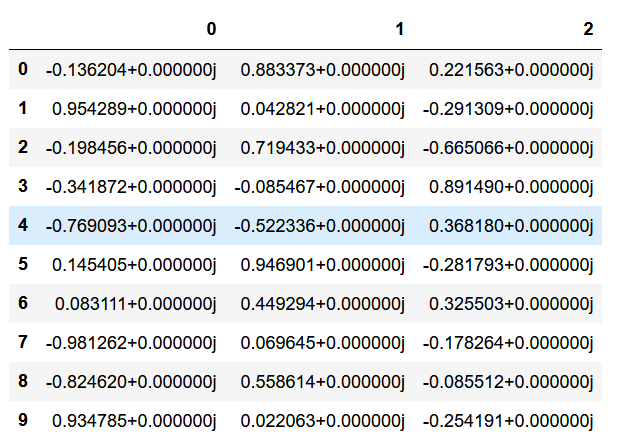计算因子得分. data=np.mat(data) #矩阵化处理factor_score=(data).dot(A) #计算因子得分factor_score=pd.DataFrame(factor_score)#数据框化factor_score.columns=['因子A','因子B','因子C'] #对因子变量进行命名factor_score#factor_score.to_excel(outputfile)#打印输出因子得分矩阵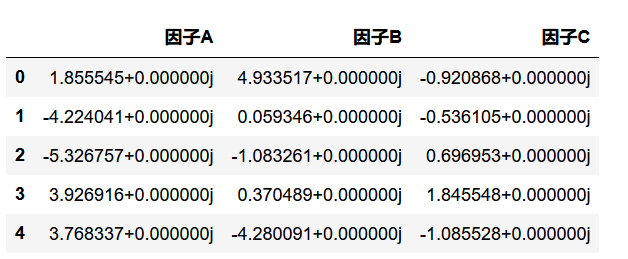喜欢分享or

展开全文• tensor、numpy、vector转换python中：**numpy -> tensor**: torch.from_numpy(ndarray)**tensor -> numpy**: tensor.numpy()123c++中：**array -> tensor**: torch::tensor(at::ArrayRef({3.1, 3.2, ...
tensor、numpy、vector转换python中：**numpy -> tensor**:  torch.from_numpy(ndarray)**tensor -> numpy**:  tensor.numpy()123c++中：**array -> tensor**:  torch::tensor(at::ArrayRef({3.1, 3.2, 3.3, ...})); 一般数组**cv::Mat -> tensor**:  torch::tensor(at::ArrayRef(img.data, img.rows * img.cols * 3)).view({img.rows, img.cols, 3}); 指针123**tensor->vector**:   vectorxxx(tensor.data(), tensor.data()+tensor.numel()); 指针直接初始化vector**tensor -> cv::Mat**:  Mat m(tensor.size(0), tensor.size(1), CV_8UC1, (void*) tensor.data());123cv::Mat 与tensor的其他转换方法：// from_blob不申请新空间，只是image.data的view。可以用.clone深拷贝。at::Tensor tensor_image = torch::from_blob(image.data, {1, 3, image.rows, image.cols}, at::kByte); //at::kByte与image的dypte一直；其它如at::kFloat等。// 之后再转其他dtypetensor_image = tensor_image.to(at::kFloat);tensor_image.data(); //这是一个float* 指针。// an exampleat::Tensor compute(at::Tensor x, at::Tensor w) {cv::Mat input(x.size(0), x.size(1), CV_32FC1, x.data());cv::Mat warp(3, 3, CV_32FC1, w.data());cv::Mat output;cv::warpPerspective(input, output, warp, {64, 64});return torch::from_blob(output.ptr(), {64, 64}).clone();}1234567891011121314151617c++ model forward 返回值返回类型为 torch::jit::IValuetorch::jit::IValue result = module->forward(inputs);1如果只有一个返回值，可以直接转tensor：auto outputs = module->forward(inputs).toTensor();1如果有多个返回值，需要先转tuple：auto outputs = module->forward(inputs).toTuple();torch::Tensor out1 = outputs->elements().toTensor();torch::Tensor out2 = outputs->elements().toTensor();123使用gpu把model和inputs都放到gpu上：std::shared_ptrmodule = torch::jit::load(argv);//put to cudamodule->to(at::kCUDA);// 注意是把tensor放到gpu，而不是vectorstd::vectorinputs;image_tensor.to(at::kCUDA)inputs.push_back(image_tensor)12345678可以指定 GPU id： to(torch::Device(torch::kCUDA, id))————————————————版权声明：本文为CSDN博主「西伯利亚的蓝眼睛」的原创文章，遵循CC 4.0 BY-SA版权协议，转载请附上原文出处链接及本声明。原文链接：https://blog.csdn.net/qq_14975217/article/details/90512374
展开全文• #-*-coding:UTF-8-*-'''Vector33D向量类关于操作符重载http://docs.python.org/reference/datamodel.html'''importmathclassVector3():def__init__(self,x,y,z):self.x=xself.y=yself.z=z#置为零向量defzero():self....
#-*- coding: UTF-8 -*-'''Vector3 3D向量类关于操作符重载 http://docs.python.org/reference/datamodel.html'''importmathclassVector3():def__init__(self,x,y,z):self.x=xself.y=yself.z=z#置为零向量defzero():self.x=0self.y=0self.z=0#"=" 赋值不支持重载#重载 "==" 操作符def__eq__(self, other):returnself.x==other.xandself.y==other.yandself.z==other.z#重载 "!=" 操作符def__ne__(self, other):returnself.x!=other.xorself.y!=other.yorself.z!=other.z#重载一元 "-" 操作符def__neg__(self):returnVector3(-self.x,-self.y,-self.z)#重载 "+" 操作符def__add__(self, other):returnVector3(self.x+other.x, self.y+other.y, self.z+other.z)#重载 "-" 操作符def__sub__(self, other):returnVector3(self.x-other.x, self.y-other.y, self.z-other.z)#重载 "*" 操作符def__mul__(self, a):returnVector3(self.x*a, self.y*a, self.z*a)#重载 "/" 操作符def__div__(self, a):if(a!=0):returnVector3(self.x/a, self.y/a, self.z/a)else:returnNone#重载 "+=" 操作符def__iadd__(self, other):self.x+=other.xself.y+=other.yself.z+=other.zreturnself#重载 "-=" 操作符def__isub__(self, other):self.x-=other.xself.y-=other.yself.z-=other.zreturnself#重载 "*=" 操作符def__imul__(self, a):self.x*=aself.y*=aself.z*=areturnself#重载 "/=" 操作符def__idiv__(self, a):if(a!=0):self.x/=aself.y/=aself.z/=areturnself#向量标准化defnormalize(self):magSq=self.x*self.x+self.y*self.y+self.z*self.zif(magSq>0):oneOverMag=1.0/math.sqrt(magSq)self.x*=oneOverMagself.y*=oneOverMagself.z*=oneOverMag#向量求模defvectorMag(self):returnmath.sqrt(self.x*self.x+self.y*self.y+self.z*self.z)#向量显示deftoString(self):print"{x:"+str(self.x)+",y:"+str(self.y)+",z:"+str(self.z)+"}"#向量点乘defdotProduct(va, vb):returnva.x*vb.x+va.y*vb.y+va.z*vb.z#向量叉乘defcrossProduct(va, vb):x=va.y*vb.z-va.z*vb.yy=va.z*vb.x-va.x*vb.zz=va.x*vb.y-va.y*vb.xreturnVector3(x,y,z)#计算两点间的距离defdistance(va, vb):dx=va.x-vb.xdy=va.y-vb.ydz=va.z-vb.zreturnmath.sqrt(dx*dx+dy*dy+dz*dz)`
展开全文• 前言在Python3.7及以上版本中，增加了一个dataclasses标准库，它主要用来做数据的容器，同时它还为开发人员预制了标准方法，并简化了类的创建过程，在这篇文章中，我们来研究一下这个标准库。我们的第一个数据类让...
• vector常用方法assign() 对Vector中的元素赋值void assign( input_iterator start, input_iterator end ); //void assign( size_type num, const TYPE &val );reserve() 设置Vector最小的元素容纳数量 函数为...
• 在人工智能浪潮之下，全民学习 Python 已成为必然趋势。Python 作为一门胶水语言，以简单的语法、良好的交互性、移植性等优势受到诸多开发者的喜爱，但要和老牌的 C++ 相较而言，谁运行的速度更快一些？相信很多...
• 运算符重载Python 关于运算符重载的规则：不能重载内置类型的运算符不能新建，只能重载某些运算符不能重载--is、and、or 和 not一元运算符__neg____pos____invert__一元运算符是返回新建对象，不能修改self....
• 本文主要讨论：解释型语言中的GPU编程ThrustRTC 项目, 以及如何在Python中使用CUDA模板库可以看作是ThrustRTC这个项目：fynv/ThrustRTC​github.com的一个引言。解释型语言与GPU编程众所周知，C++ 是CUDA的默认语言...
• 全文共2607字，预计学习时长14分钟图源：unsplash数据类适用于Python3.7或更高版本，它不仅可以用作数据容器，还可以编写样板代码，简化创建类的过程。创建第一个数据类创建一个数据类，该数据类表示三维坐标系中的...
• 全文共3168字，预计学习时长8分钟图源：Google本教程将介绍如何使用机器学习技术(如word2vec和余弦相似度等)，在Python中用几行代码制作抄袭检测器。搭建完成后，抄袭检测器将会从文件中载入学生们的作业，然后通过...
• 字典的操作字符串操作常用的字符串处理name = 'vector'print(name.capitalize()) # 首字母大写print(name.count('e')) # 判断字符e在字符串中有多少个print(name.center(50,'-')) # 一共打印50个字符,将vector放在...
• I get an error I can't make sense of trying to wrap a rather simple c++ class via Boost.Python.First, the class:#include #include #include #include class token {public:typedef boost::shared_ptr ptr_ty...
• 使用std :: for_each和lambda可以做一些有趣的事情。#include #include int main(){int array[] = {1,2,3,4};std::for_each(std::begin(array), std::end(array),[&std::cout, sep=' '](int x) mutable {out <...
• Vector(7,8) 方法重写 何为方法重写，为啥需要重写呢，因为父类的方法不合适我们，也就是不满足我们的需求。 uper() 函数是用于调用父类(超类)的一个方法。 输出结果为： 调用子类方法调用父类方法 如果你处于想学...
• str = '你好' #假设当前为utf-8 注意:Python3里面的默认编码为Unicode str = str.decode('utf-8') #将utf-8转换为Unicode str = str.encode('gbk') #将Unicode转换为gbk print(str) 如果该文件为gbk，同时Python3的...
• python 语言中，解决这道题，使用暴力方法一定会遇到超时的问题，为此使用类似hash表的‘巧妙'一点的O(n)的方法。Loading...​leetcode.com题目描述：给定一个整数数组和一个整数k，你需要找到该数组中和为k的连续...
• } 错误说明： error C2664: “std::vector<:ssbody>>::vector(std::initializer_list,std::string>>,const std::allocator<_ty> &)”: 无法将参数 1 从“std::pair,const char *>”转换为“const std::allocator...
• 现在的问题是，我无法让函数正确返回这个 vector，里面是 4 个二进制字符串，win 上写入文件大小大约在 33-70kb因为直接返回 vector会出现 utf-8 无法识别 0x01 之类的报错，然后用了 py::bytes 这个方法我尝试了用...
• 介绍今天开始一个新的系列，这个系列的目标是用python在不使用任何第三方库的情况下去实现各类机器学习或者深度学习的算法。之所以会有这种想法是因为每当我想提高编程技巧的时候，我总希望能够做一些简单又有趣的小...
• 贪吃蛇、迷宫、吃豆人、扫雷、Flappy Bird...这些游戏可以是非常经典，甚至伴随着很多人的童年回忆。那么，你是否想过自己开发一...那么，今天要介绍的这款Python项目就可以轻松实现你成为游戏开发者的想法，实现前...
• Free Python Games非常适合学生，它不仅具有高度的组织性和灵活性，而且能够激发人们探索和理解能力。--Terri FurtonFree Python Games在轻松的环境中把游戏和学习结合在一起，从而减轻了编程过程中的压力。--Brett ...
• Code #-*-coding:UTF-8-*- ''' Vector33D向量类 ...关于操作符重载http://docs.python.org/reference/datamodel.html ''' importmath classVector3(): def__init__(self,x,y,z): self.x=x ...
• 对于前述的tensor_types vector，初始化里面的每一个python type元素(如torch.FloatTensor, torch.DoubleTensor等等)；然后将这些类型添加到其对应的python module上，像下面这样： ByteTensor-->torch CharTensor-...
• 今天使用boost.python封装一个vector，出现了错误。 代码大致如下： class Point { public: Point( double xt , double yt ):x(xt) , y(yt){} double getX(){ return x; } double getY(){ return y; } ...microsoft class algorithm
• ## python实现 vector

万次阅读 2018-09-07 17:25:17
#_*_coding:utf-8_*_ list = [] list1 = [] for i in range(0,6): for j in range(0,i+1): list.append(j) list1.append(list) list = [] print(list1)
• 前言C++和Python都是当前最流行的语言之一，其强大的功能毋庸置疑，它们分别在不同甚至相同的领域发挥着至关重要的作用。C++是底层开发中起着中流砥柱的作用，屹立多年依然势头强劲不露颓势。而Python在最近大热的...
• Python Wordvector (二) import jieba import pandas as pd txt = open("hlm.txt", "r", encoding="gb18030").read() from gensim.models.word2vec import Word2Vec ......

# pythonvectorpython 订阅